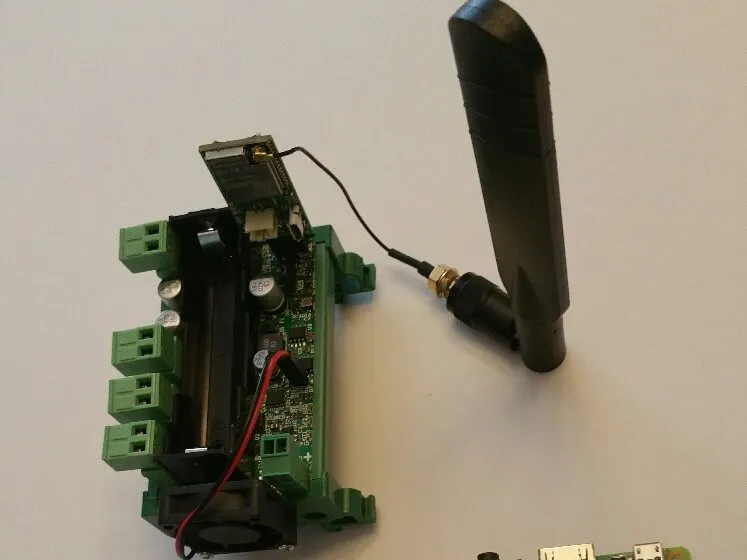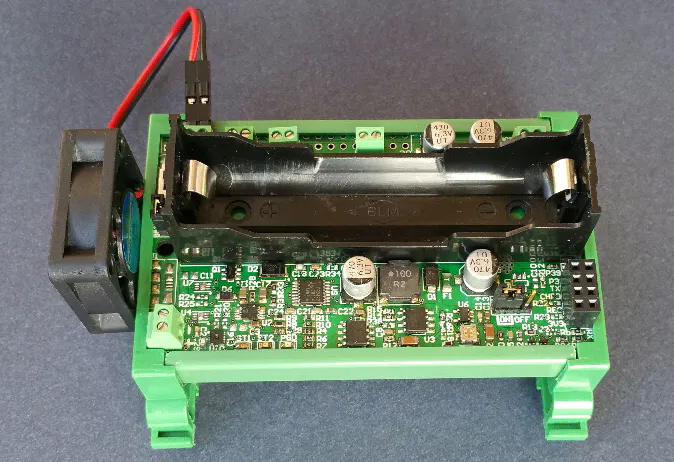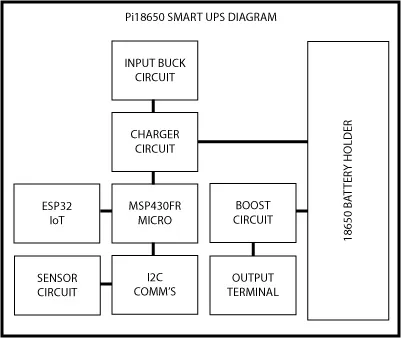Published

# Pi18650 SMART UPS - IoT

A smart mobile UPS for Raspberry Pi and IoT.

IntermediateProtip1 hour438## Custom parts and enclosures

### DIN Rail Housing

Used for mounting to Din Rail.## Schematics

### Pi18650 SMART UPS - Diagram

Block diagram of system components.## Code

### Pi18650 SMART UPS - Sensor Readings

Python
This code is used to read the temperature and humidity data from the SMART UPS and is written in Python and can be run on the Raspberry Pi.
```import io
import fcntl
import struct
import time

I2C_SLAVE=0x0703

class i2c:

def __init__(self, device, bus):

self.fr = io.open("/dev/i2c-"+str(bus), "rb", buffering=0)
self.fw = io.open("/dev/i2c-"+str(bus), "wb", buffering=0)

fcntl.ioctl(self.fr, I2C_SLAVE, device)
fcntl.ioctl(self.fw, I2C_SLAVE, device)

def write(self, bytes):
self.fw.write(bytes)

def close(self):
self.fw.close()
self.fr.close()

def get_int(self, var_slice):

ms = memoryview(var_slice)
var_list = map(ord,ms)
#print("bat_list: ", bat_list)
var_int = var_list*256 + var_list

return var_int

testpoll = i2c(0x48,1)
samples = 10
bat_avg = 0
hum_avg = 0
temp_avg = 0
sample_delay = 0.010

for i in range(samples):
print("result: ", result)

bat = result[:2]
hum = result[2:4]
temp = result[4:6]
temp1 = result[6:8]
temp2 = result[-2:]

for j in range(3):
if j == 0:
res = testpoll.get_int(bat)
bat_avg = bat_avg + res

if j == 1:
res1 = testpoll.get_int(hum)
hum_avg  = hum_avg + res1

if j == 2:
res2 = testpoll.get_int(temp)
temp_avg  = temp_avg + res2

result = '0'
res = 0
res1 = 0
res2 = 0
time.sleep(sample_delay)

#m = memoryview(bat)
#bat_list = map(ord,m)
#print("bat_list: ", bat_list)

#Battery Level calculation---------------
#bat_int = bat_list*256 + bat_list
#print("bat_int: ", bat_int)

bat_avg_int = bat_avg / samples
battery = (float(bat_avg_int) * 0.00322) / 0.7674
print "Battery: ", battery ,"V"
#----------------------------------------

#m1 = memoryview(hum)
#hum_list = map(ord,m1)
#print("hum_list: ", hum_list)

#Humidity calculation--------------------
#hum_int = hum_list*256 + hum_list
#print("hum_int: ", hum_int)

humidity = 0.0
hum_avg_int = hum_avg / samples
humidity = float(-12.5) + (float(125) * (float(hum_avg_int) / float(1024)))
humidity  = round(humidity,2)
print "Humidity: ", humidity ,"%"
#----------------------------------------

#m2 = memoryview(temp)
#temp_list = map(ord,m2)
#print("temp_list: ", temp_list)

#Temperature calculation - Deg C-----------
#temp_int = temp_list*256 + temp_list
#print("temp_int: ", temp_int)

temperature = 0.0
temp_avg_int = temp_avg / samples
temperature = float(-66.875) + (float(218.75) * (float(temp_avg_int) / float(1024)))
temperature = round(temperature,2)
print "Temperature: ", temperature ,"C"
#-----------------------------------------

#Temperature calculation - Deg F-----------
#temp_int = temp_list*256 + temp_list
#print("temp_int: ", temp_int)

temperatureF = 0.0
temp_avg_int = temp_avg / samples
temperatureF = float(-88.375) + (float(393.75) * (float(temp_avg_int) / float(1024)))
temperatureF = round(temperatureF,2)
print"Temperature: ", temperatureF ,"F"
#-----------------------------------------

#dev.write("\x2D\x00") # POWER_CTL reset

testpoll.close()
```

## Credits

### Mark Chin

5 projects • 10 followers
Entrepreneur and Innovator of embedded systems, RFID and IoT.